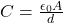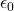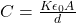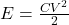An air-filled parallel-plate capacitor is connected to a battery and allowed to charge up. Now a slab of dielectric material is placed betwe

Question

An air-filled parallel-plate capacitor is connected to a battery and allowed to charge up. Now a slab of dielectric material is placed between the plates of the capacitor while the capacitor is still connected to the battery.

After this is done, we find that :

a. the charge on the capacitor had increased.

b. the energy stored in the capacitor had decreased.

c. the voltage across the capacitor had increased.

d. the charge on the capacitor had not changed.

e. None of these choice are true.

in progress 0
5 months 2021-08-16T04:31:08+00:00 1 Answers 87 views 0

The charge on the capacitor had increased

Explanation:

The expression for the capacitance of an air-filled parallel-plate capacitor is as follows as;Here, C is the capacitance,is the permittivity of free space, A is the area and d is the distance between the parallel plate capacitor.

When a slab of dielectric material is placed between the plates of the capacitor then the expression for the capacitance is as follows;Here, K is the dielectric constant.

In the given problem, a slab is inserted between the plates of the capacitor then the capacitance of the capacitor will increase in this case. Therefore, the option (a) is true.

The expression for the charge stored in the capacitor is as;

Q= CV

Here, Q is the charge and V is the potential.

The charge will also increase in this case as the charge stored in the capacitor is directly proportional to the capacitance. Therefore, the option (d) is not true.

The expression for the energy stored in the capacitor is as follows;The voltage is constant in the given problem but the capacitance increases then the energy stored in the capacitor will increase. Therefore, the option (b) is not true.

The voltage across the capacitor will remain same as the capacitor is still connected to the battery. Therefore, the option (c) is not true.

Therefore, only option (a) is true.